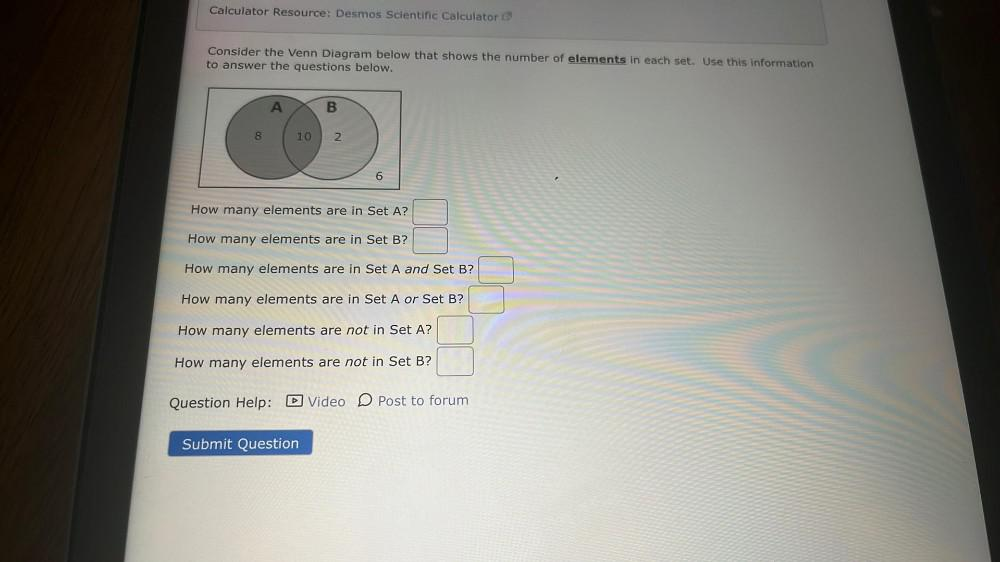Question:

# Calculator Resource: Desmos Scientific Calculator Consider the Venn Diagram below that shows the number of elements in each set.Calculator Resource: Desmos Scientific Calculator Consider the Venn Diagram below that shows the number of elements in each set. Use this information to answer the questions below. A B 8 10 2 6 How many elements are in Set A? How many elements are in Set B? How many elements are in Set A and Set B? How many elements are in Set A or Set B? How many elements are not in Set A? How many elements are not in Set B? Question Help: D Video D Post to forum Submit Question